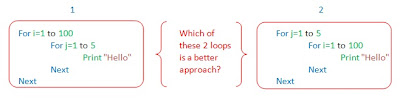## Tuesday, June 7, 2011

### Nested loops : Best practices

Consider the following two examples:Click on the image to enlarge

I have 3 questions for you now.

1) Will both the loops take the same time to execute?
2) Which is a better programming approach?

75% of the programmers would say, there will not be any changes, both the loops will execute identical. Who knows you may also think that way, I bet you are gonna change that opinon by end of this article. Or atleast know why we follow something as a ‘best practice’

Let us analyse both the situations. In both cases there are 3 execuatable statements viz two for loops and one Print statement (Lines 1,2 &3 shown below).

Assumption : Let us assume that every executable statement takes 1 second to execute (it is easy to calculate stuff particularly multiplication :) )

Let us analyse the first situation:

Let us go line by line,

Line 1 (For i=1 to 100) : This will be executed for 100 positive conditions and 1 negative condition. Total 101 executions, there by taking 101 seconds (This is why I assumed execution time as 1 sec/statement)
Execution time for line 1 = 101 seconds

Line 2 (For j=1 to 5) : This statement will be executed for 5 positive conditions and 1 negative condition. Total 6 executions for every positve conditions of line 1, which will be 100. So making it 100x6 executions which will be 600.
Execution time for line 2 = 600

Line 3 (Print “Hello”) : For statement will be executed for every positive condition of Line 1 and Line 2. Therefore, 100x5 times it will be executed.
Execution time for line 3 = 500

Therfore the total execution time for first situation = 101 + 600 + 500 = 1201 seconds

Let us now analyse the second situation:

Let us again go line by line,

Line 1 (For j=1 to 5) : This will be executed for 5 positive conditions and 1 negative condition. Total 6 executions, there by taking 6 seconds
Execution time for line 1 = 6 seconds

Line 2 (For i=1 to 100) : This statement will be executed for 100 positive conditions and 1 negative condition. Total 101 executions for every positve conditions of line 1, which will be 5. So making it 5x101 executions which will be 505.
Execution time for line 2 = 505

Line 3 (Print “Hello”) : For statement will be executed for every positive condition of Line 1 and Line 2. Therefore, 5x100 times it will be executed.
Execution time for line 3 = 500

Here the total execution time for 2nd situation = 6 + 505 + 500 = 1011 seconds.

Did you ever realize this??? Yes, we should have the lesser iteration loop in the outer and more iterations loop inner. I had goosebumps when I came to know about it the first time.

Also, read QTP best practices and QTP coding standards and guidelines.

1.Great work !! Very hard nested if and you slove normaly ! Thanks for sharing.

2.Oh, haha.

Either (100 + 1) x 5 = 101 x 5
OR
(5+1) x 100 = 6 x 100.

Wait, why did you add the extra false on the first iteration, of 5? and the 101? 5 iterations is 5 iterations, it's not a while loop, breaking entire loop once hitting false.

What if the T/F ratio was different? The more breaks on the first statement mean less iterations on the second. Making the difference between the two closer to the same. I would imagine, that eventually, having the greater iteration, with enough false matches, would decrease the amount of loops.

Assuming that extra one is in there, which I don't really get:

100 iterations on the outside, 50 false, 50 true:

101 = 101 Line 1
50x6=300 Line 2
50x5=250 Line 3
651

5 iterations on outside, 100 on inside, 50 false

6
5x101=505
5x50=250
761

Thus having the 100 iterations on the outside shorter - if I did my math/logic properly. Where the two would be equal, I don't feel like figuring out.

Either way, which loop is inside/outside is often not a choice. Or, you sacrifice intuitive logic for a few seconds, which in mind mind is only worth it in some situations.

Well, I'm generally not building huge applications with an exessive amount of nested loops, so I'll just leave this as an academic tidbit for future reference. Cool though!

3.You have really done a great work to share the hidden art of the great man. It is really a nice work by them. Thanks a lot for this
Audi A3 Turbocharger

4.Goose bumps, huh? WOW - I thought I was the only person that geeky!! LOL
I mean that as a complete compliment.
Have a great day and thanks for sharing these useful tidbits.

5.